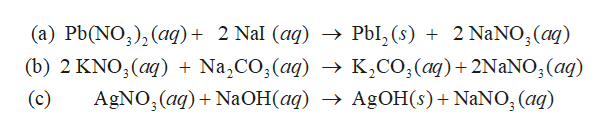# Write a balanced chemical equation for the double replacement reactions listed below. Use the solubility rules in the introduction section of this experiment to predict whether a double replacement reaction will occur and a solid is formed. For full credit, include the states of matter in the balanced chemical equarion. If there is no reaction, write NR after the arrow. a.) _____ Pb(NO3)2(aq) + _____ NaI(aq) ---> ________________________b.) ______ KNO3(aq) + ________ Na2CO3(aq) ----> ___________________c.) ______ AgNO3(aq) + _______ NaOH(aq) ---> _____________________d.) ______ HNO3(aq) + ________ K2CO3(aq) ---> _______________

Question
67 views

Write a balanced chemical equation for the double replacement reactions listed below. Use the solubility rules in the introduction section of this experiment to predict whether a double replacement reaction will occur and a solid is formed. For full credit, include the states of matter in the balanced chemical equarion. If there is no reaction, write NR after the arrow.

a.) _____ Pb(NO3)2(aq) + _____ NaI(aq) ---> ________________________

b.) ______ KNO3(aq) + ________ Na2CO3(aq) ----> ___________________

c.) ______ AgNO3(aq) + _______ NaOH(aq) ---> _____________________

d.) ______ HNO3(aq) + ________ K2CO3(aq) ---> _______________

check_circle

star
star
star
star
star
1 Rating
Step 1

Since you have posted a question with multiple sub-parts, we will solve first three subparts for you. To get the remaining sub-part solved please repost the complete question and mention the sub-parts to be solved

Step 2

The double replacement reactions are represented as below. The equations are balanced by balancing...help_outlineImage Transcriptionclose(a) Pb(NO)2 (aq) 2 Nal (aq) -> Pbl, (s) 2 NaNO,(aq) (b) 2 KNO,(aq) + Na,CO2(aq) -> K,CO;(aq)+2NaNO,(aq) AgNO (aq) NaOH(aq) > A^OH(s)+NaNO, (aq) (c) fullscreen

### Want to see the full answer?

See Solution

#### Want to see this answer and more?

Solutions are written by subject experts who are available 24/7. Questions are typically answered within 1 hour.*

See Solution
*Response times may vary by subject and question.
Tagged in

### Chemistry